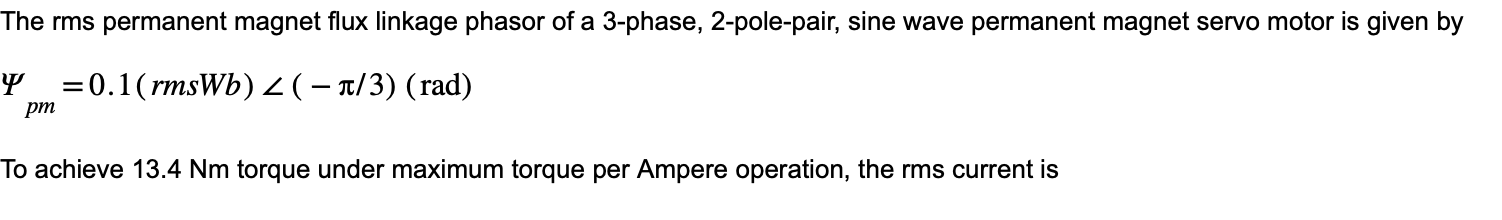# Question Solved1 Answer​​​​​​​ The rms permanent magnet flux linkage phasor of a 3-phase, 2-pole-pair, sine wave permanent magnet servo motor is given by $\Psi_{p m}=0.1(\mathrm{rms} W b) \angle(-\pi / 3)(\mathrm{rad})$ To achieve $$13.4 \mathrm{Nm}$$ torque under maximum torque per Ampere operation, the rms current isXQ520E The Asker · Electrical Engineering​​​​​​​

Transcribed Image Text: The rms permanent magnet flux linkage phasor of a 3-phase, 2-pole-pair, sine wave permanent magnet servo motor is given by $\Psi_{p m}=0.1(\mathrm{rms} W b) \angle(-\pi / 3)(\mathrm{rad})$ To achieve $$13.4 \mathrm{Nm}$$ torque under maximum torque per Ampere operation, the rms current is
More
Transcribed Image Text: The rms permanent magnet flux linkage phasor of a 3-phase, 2-pole-pair, sine wave permanent magnet servo motor is given by $\Psi_{p m}=0.1(\mathrm{rms} W b) \angle(-\pi / 3)(\mathrm{rad})$ To achieve $$13.4 \mathrm{Nm}$$ torque under maximum torque per Ampere operation, the rms current is